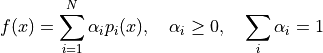# Create a mixture of PDFs¶

In this example we are going to build a distribution whose PDF is defined by a linear combination of probability density functions:The weigths are automatically normalized.

It is also possible to create a mixture of copulas.

import openturns as ot
import openturns.viewer as viewer
from matplotlib import pylab as plt

ot.Log.Show(ot.Log.NONE)


create a collection of distribution and the associated weights

distributions = [
ot.Triangular(1.0, 2.0, 4.0),
ot.Normal(-1.0, 1.0),
ot.Uniform(5.0, 6.0),
]
weights = [0.4, 1.0, 0.2]


create the mixture

distribution = ot.Mixture(distributions, weights)
print(distribution)

Mixture((w = 0.25, d = Triangular(a = 1, m = 2, b = 4)), (w = 0.625, d = Normal(mu = -1, sigma = 1)), (w = 0.125, d = Uniform(a = 5, b = 6)))


draw PDF

graph = distribution.drawPDF()
view = viewer.View(graph)define a list of copulas and the associated weights

copulas = [ot.GumbelCopula(4.5), ot.ClaytonCopula(2.3)]
weights = [0.2, 0.8]


create a mixture of copulas

distribution = ot.Mixture(copulas, weights)
print(distribution)

Mixture((w = 0.2, d = GumbelCopula(theta = 4.5)), (w = 0.8, d = ClaytonCopula(theta = 2.3)))


draw PDF

graph = distribution.drawPDF()
view = viewer.View(graph)
plt.show()